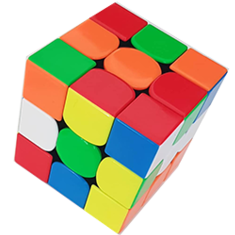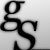# Export information 3DPolyLine To Chainage And Elevation## Recommended PostsI Want Required lisp Export Data of 3DPolyLine To CSV "Chainage" And "Elevation"

This lisp Proposed use for Cross Section.

##### Share on other sitesHere is a pline co-ords example it will make a list of x y z points its up to you what you want to do next.

```(defun getcoords (ent)
(vlax-safearray->list
(vlax-variant-value
(vlax-get-property
(vlax-ename->vla-object ent)
"Coordinates"
)
)
)
)

(defun co-ords2xy (I numb xy)
; convert now to a list of xy as co-ords are x y x y x y if 3d x y z x y z
(setq numb (/ (length co-ords) 3))
(setq I 0)
(repeat numb
(setq xy (list (nth i co-ords)(nth (+ I 1) co-ords)(nth (+ I 2) co-ords) ))
(setq co-ordsxy (cons xy co-ordsxy))
(setq I (+ I 2))
)
)

; program starts here

(setq co-ords (getcoords (car (entsel "\nplease pick pline"))))
(co-ords2xy)

```

##### Share on other sitesHere is a pline co-ords example it will make a list of x y z points its up to you what you want to do next.

```(defun getcoords (ent)
(vlax-safearray->list
(vlax-variant-value
(vlax-get-property
(vlax-ename->vla-object ent)
"Coordinates"
)
)
)
)

(defun co-ords2xy (I numb xy)
; convert now to a list of xy as co-ords are x y x y x y if 3d x y z x y z
(setq numb (/ (length co-ords) 3))
(setq I 0)
(repeat numb
(setq xy (list (nth i co-ords)(nth (+ I 1) co-ords)(nth (+ I 2) co-ords) ))
(setq co-ordsxy (cons xy co-ordsxy))
(setq I (+ I 2))
)
)

; program starts here

(setq co-ords (getcoords (car (entsel "\nplease pick pline"))))
(co-ords2xy)

```

Thank you sir for replay, But I want lisp file 3Dpolyline to Distance And E

Total Topography Center line.dwg

Required Sample.CSV

##### Share on other sitesNot sure if this is what you need sanju2323

```(Defun c:demo (/ csvfile cnt sn i a)
(if (and
(= (getvar 'DwgTitled) 1)
(setq ss (ssget "_:S:E" '((0 . "POLYLINE"))))
)
(progn
(setq csvfile_ (strcat (getvar 'DWgprefix)
(vl-filename-base (getvar 'dwgname))
".csv"
)
)
(setq csvfile (open csvfile_ "w"))
(write-line "Distance,Elevation" csvfile)
(setq cnt 0 sn  (ssname ss 0))
(setq param (fix (vlax-curve-getEndParam sn)))
(repeat	(1+ param)
(write-line
(strcat
(rtos (vlax-curve-getdistatparam sn cnt) 2
","
(rtos (last (vlax-curve-getpointatparam sn cnt)) 2 4)
)
csvfile
)
(setq cnt (1+ cnt))
)
(close csvfile)
)
)
)```

##### Share on other sitesNot sure if this is what you need sanju2323

```(Defun c:demo (/ csvfile cnt sn i a)
(if (and
(= (getvar 'DwgTitled) 1)
(setq ss (ssget "_:S:E" '((0 . "POLYLINE"))))
)
(progn
(setq csvfile_ (strcat (getvar 'DWgprefix)
(vl-filename-base (getvar 'dwgname))
".csv"
)
)
(setq csvfile (open csvfile_ "w"))
(write-line "Distance,Elevation" csvfile)
(setq cnt 0 sn  (ssname ss 0))
(setq param (fix (vlax-curve-getEndParam sn)))
(repeat    (1+ param)
(write-line
(strcat
(rtos (vlax-curve-getdistatparam sn cnt) 2
","
(rtos (last (vlax-curve-getpointatparam sn cnt)) 2 4)
)
csvfile
)
(setq cnt (1+ cnt))
)
(close csvfile)
)
)
)```

This Lisp Error shown as

" ; error: no function definition: VLAX-CURVE-GETENDPARAM"

##### Share on other sites@ Sanju Enter (vl-load-com) at the command Prompt

and Try again with pBe Codes

##### Share on other sitesNot sure if this is what you need sanju2323

Maybe the OP's request is for horizontal distances (see posted dwg/csv).```;Copyright  © pBe
(Defun c:demo (/ csvfile cnt sn i a hyp h progr)
(if (and
(= (getvar 'DwgTitled) 1)
(setq ss (ssget "_:S:E" '((0 . "POLYLINE")(-4 . "&=") (70 . )))
)
(progn
(setq csvfile_
(strcat
(getvar 'DWgprefix)
(vl-filename-base (getvar 'dwgname))
".csv"
)
)
(setq csvfile (open csvfile_ "w"))
(write-line "Distance,Elevation" csvfile)
(setq cnt 0 sn  (ssname ss 0) progr 0.)
(setq param (fix (vlax-curve-getEndParam sn)))
(repeat (1+ param)
(if (> cnt 0)
(progn
(setq hyp (- (vlax-curve-getdistatparam sn cnt) (vlax-curve-getdistatparam sn (- cnt 1))))
(setq h (- (last (vlax-curve-getpointatparam sn cnt)) (last (vlax-curve-getpointatparam sn (- cnt 1)))))
(setq progr (+ progr (sqrt (- (* hyp hyp) (* h h)))))
)
(progn
(setq hyp (vlax-curve-getdistatparam sn cnt))
(setq h (last (vlax-curve-getpointatparam sn cnt)))
(setq progr 0.)
)
)
(write-line
(strcat
(rtos progr 2 7)
","
(rtos (last (vlax-curve-getpointatparam sn cnt)) 2 4)
)
csvfile
)
(setq cnt (1+ cnt))
)
(close csvfile)
)
)
(princ)
)```

##### Share on other sitesMaybe the OP's request is for horizontal distances (see posted dwg/csv).. Whoa! You are right GP_

```...
(progn
(setq hyp (- (vlax-curve-getdistatparam sn cnt) (vlax-curve-getdistatparam sn (- cnt 1))))
(setq h (- (last (vlax-curve-getpointatparam sn cnt)) (last (vlax-curve-getpointatparam sn (- cnt 1)))))
(setq progr (+ progr (sqrt (- (* hyp hyp) (* h h)))))
....
```

Nice solutionHere's another approach

```(Defun c:demo2  (/ csvfile cnt sn i a b)
(if (and
(= (getvar 'DwgTitled) 1)
(setq ss (ssget "_:S:E" '((0 . "POLYLINE")(-4 . "&=") (70 . )))
)
(progn
(setq csvfile_
(strcat (getvar 'DWgprefix)
(vl-filename-base
(getvar 'dwgname))
".csv"
)
)
(setq csvfile (open csvfile_ "w"))
(write-line "Distance,Elevation" csvfile)
(setq cnt 0.0
a   nil
b   nil)
(setq pts (vlax-get
(vlax-ename->vla-object (ssname ss 0))
'Coordinates))
(while pts
(setq a   (cons (list (car pts) (cadr pts))
a)
pts (cdddr pts)))
(Setq b    (reverse b) p '(0.0 0.0))
(mapcar (function (lambda (p1 p2 z)
(write-line  (Strcat
(rtos (setq cnt  (+ (distance p1 p2) cnt)) 2 7)
","
(rtos z 2 4)
)
csvfile
)
)
)
(cons p (setq a (reverse a)))
(cons p (cdr a))
b)
(close csvfile)
)
)
(princ)
)
```

Edited by pBe
##### Share on other sitesThank to all replays

##### Share on other sitesHere's another approach...

Excellent.##### Share on other sites

• 6 years later...```(Defun c:demo2  (/ csvfile cnt sn i a b)
(if (and
(= (getvar 'DwgTitled) 1)
(setq ss (ssget "_:S:E" '((0 . "POLYLINE")(-4 . "&=") (70 . )))
)
(progn
(setq csvfile_
(strcat (getvar 'DWgprefix)
(vl-filename-base
(getvar 'dwgname))
".csv"
)
)
(setq csvfile (open csvfile_ "w"))
(write-line "Distance,Elevation" csvfile)
(setq cnt 0.0
a   nil
b   nil)
(setq pts (vlax-get
(vlax-ename->vla-object (ssname ss 0))
'Coordinates))
(while pts
(setq a   (cons (list (car pts) (cadr pts))
a)
pts (cdddr pts)))
(Setq b    (reverse b) p '(0.0 0.0))
(mapcar (function (lambda (p1 p2 z)
(write-line  (Strcat
(rtos (setq cnt  (+ (distance p1 p2) cnt)) 2 7)
","
(rtos z 2 4)
)
csvfile
)
)
)
(cons p (setq a (reverse a)))
(cons p (cdr a))
b)
(close csvfile)
)
)
(princ)
)

I get error

; error: extra right paren on input
_\$

##### Share on other sites```     (princ)
)
) ; this is missing code not tested further

##### Share on other sites; error: extra right paren on input
_1\$

Even same error after adding missing  ")"

```(startapp "notepad" csvfile_)
)
)
(princ)
)
)
##### Share on other sites(setq ss (ssget "_:S:E" '((0 . "POLYLINE")(-4 . "&=") (70 . ? ))))

##### Share on other sitesThis is likely a byproduct of the "upgrade" to the forum software - I imagine the code should read:

`(setq ss (ssget "_:S:E" '((0 . "POLYLINE")(-4 . "&=") (70 . 8))))`

When the forum software was updated, all occurrences of "8)" in all code snippets were removed.

##### Share on other sitesNice catch Lee and GP the not so obvious.

## Join the conversation

You can post now and register later. If you have an account, sign in now to post with your account.
Note: Your post will require moderator approval before it will be visible.Unfortunately, your content contains terms that we do not allow. Please edit your content to remove the highlighted words below.

×   Pasted as rich text.   Restore formatting

Only 75 emoji are allowed.